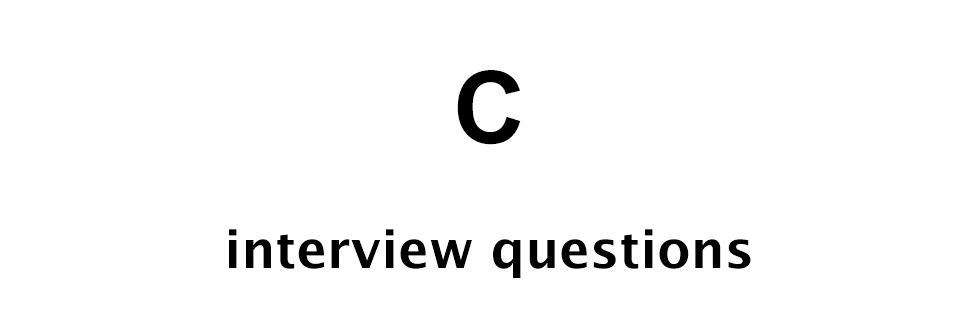# C Interview Questions - Set 4

C Programming Interview Questions## Q1: Define Array

An array is a collection of similar type of elements stored in a sequential memory location.

## Q2: Create an array of 5 integer numbers and print their mean in C programming language

We can write the following C program.

``````#include <stdio.h>

int main() {

int arr;
int i;
int sum = 0;
float mean;

// get data
printf("Enter 5 integer numbers: ");
for (i = 0; i < 5; i++) {
scanf("%d", &arr[i]);
sum += arr[i];
}

mean = sum / 5.0;

printf("Mean: %f\n", mean);

return 0;

}
``````

NOTE! In the above code we are using 5.0 in the line `mean = sum / 0.5;` to get the decimal part.

## Q3: What is a pointer?

A pointer is a special variable that holds the address of another variable of similar data type.

Example:

``````// integer variable
int i = 10;

// integer pointer variable
int *ptr;

// assigning the address of i to ptr
ptr = &i;
``````

## Q4: What is pointer to pointer?

When a pointer holds the address of another pointer then we call it pointer to pointer.

Example:

``````// integer variable
int i = 10;

// integer pointer variable
int *ptr;

// another integer pointer
int *ptr2;

// assigning the address of i to ptr
ptr = &i;

// assigning the address of ptr to ptr2
ptr2 = &ptr;
``````

## Q5: What is static and dynamic memory allocation in C?

In static memory allocation, the memory location is allocated during compile time and it can't be modified later during code execution.

Example of static memory allocation is an array.

Whereas, in dynamic memory allocation, the memory location is allocated during the program execution.

Example of dynamic memory allocation is a linked list.

## Q6: What is the full form of ANSI?

ANSI stands for American National Standard Institute.

## Q7: Explain type casting in C with an example

Conversion of one data type into another is called type casting in C.

In the following example we are type casting an integer value to a float value.

``````// integer variable
int i = 10;

// float variable
float f;

// type casting
f = (float) i;
``````

## Q8: Write C program to swap value of two integer variables without using a third variable

We can write the following code to achieve the required result.

``````#include <stdio.h>

int main() {

int x = 10;
int y = 20;

printf("Before: x = %d and y = %d\n", x, y);

x = x + y;	// 10 + 20 = 30
y = x - y;	// 30 - 20 = 10
x = x - y;	// 30 - 10 = 20

printf("After: x = %d and y = %d\n", x, y);

return 0;

}
``````

## Q9: Write a program in C to reverse a given string

We can write the following code to reverse a given string in C programming language.

``````#include <stdio.h>
#include <string.h>

int main() {

char *str, ch;
int len, i;

// get string
gets(str);

// find length
len = strlen(str);

// reverse
for (i = 0; i < len/2; i++) {
ch = str[len - i - 1];
str[len - i - 1] = str[i];
str[i] = ch;
}

// output
puts(str);

return 0;

}
``````

## Q10: Write C code to implement strlen function

The `strlen` C library function returns the number of characters in a given string.

We can write the following code to implement the strlen function.

``````#include <stdio.h>

int my_strlen(const char*);

int main() {

char *str;
int len;

// get string
gets(str);

// find length
len = my_strlen(str);

// output
printf("Length of the string: %d\n", len);

return 0;

}

int my_strlen(const char *str) {
int i;
for (i = 0; str[i] != '\0'; i++) {
i++;
}
return (i > 0) ? i - 1 : i;
}
``````